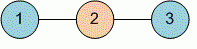Competitions

# Cheating away!

During the quiz, Professor Floyd noticed that some students exchange notes. Initially he wanted to give them all fail, but that day the professor was kind, and therefore he decided to divide the students into two groups: cheating group and those who give to copy, and give the fail only to the first group.

The professor wrote down all the pairs of students who exchanged notes. Determine will he be able to divide students into two groups so that any exchange of notes is carried out from a student of one group to a student of another group.

#### Input

First line contains two numbers n and m - the number of students and the number of student pairs who exchange notes (1n100, 0m ≤ (n * (n - 1)) / 2). Next m lines describe the pairs of students: two different of students exchanging notes (the numbering of students starts from 1). Each pair of students is listed no more than once.

#### Output

Print the answer to the Professor Floyd's problem. If you can divide students into two groups, output "YES", otherwise output "NO".Time limit 1 second
Memory limit 128 MiB
Input example #1
3 2
1 2
2 3

Output example #1
YES